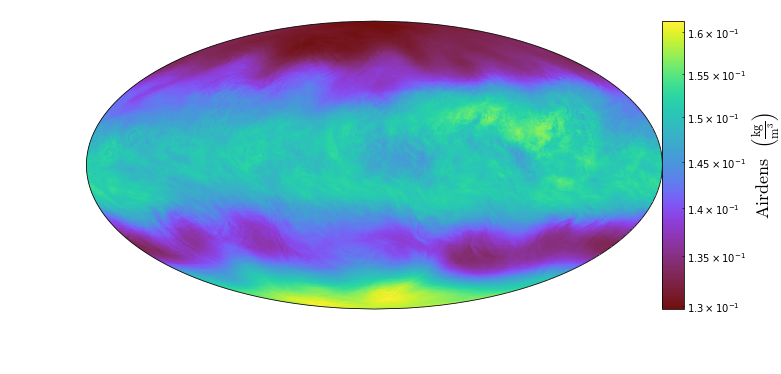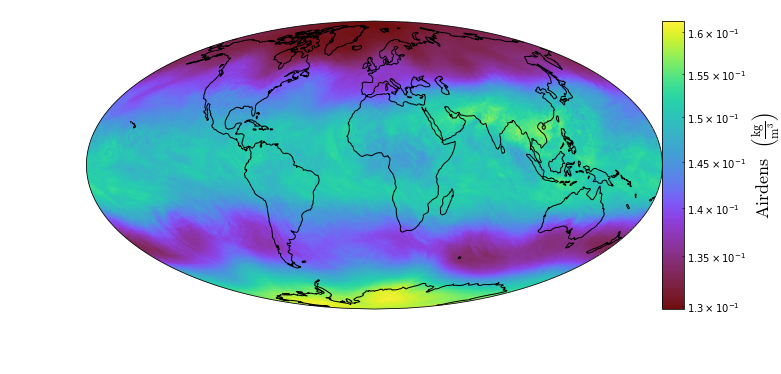# Using Geographic Transforms and Projections¶

This feature requires cartopy to be installed on your machine. This installation varies by machine. For more detailed instructions on how to do this, please refer to Installing Cartopy.

Notebook

# Geographic Transforms and Projections¶

For this analysis we'll be loading some global climate data into yt. A frontend does not exist for this dataset yet, so we'll load it in as a uniform grid with netcdf4.

In :
import yt
import numpy as np
import re
import netCDF4 as nc4
import os

In :
def get_data_path(arg):
if os.path.exists(arg):
return arg
else:
return os.path.join(yt.config.ytcfg.get("yt", "test_data_dir"), arg)

In :
n = nc4.Dataset(get_data_path("geos/GEOS.fp.asm.inst3_3d_aer_Nv.20180822_0900.V01.nc4"))


Using the loaded data we'll fill arrays with the data dimensions and limits. We'll also rename vertical level to altitude to be clearer.

In :
dims = []
sizes = []
bbox = []
ndims = len(n.dimensions)
for dim in n.dimensions.keys():
size = n.variables[dim].size
if size > 1:
bbox.append([n.variables[dim][:].min(),
n.variables[dim][:].max()])
dims.append(n.variables[dim].long_name)
sizes.append(size)
dims.reverse()   # Fortran ordering
sizes.reverse()
bbox.reverse()
dims = [f.replace('vertical level', 'altitude') for f in dims]
bbox = np.array(bbox)


We'll also load the data into a container dictionary and create a lookup for the short to the long names

In :
w_regex = re.compile(r'([a-zA-Z]+)(.*)')
def regex_parser(s):
try:
return "**".join(filter(None, w_regex.search(s).groups()))
except AttributeError:
return s

In :
data = {}
names = {}
for field, d in n.variables.items():
if d.ndim != ndims:
continue
units = n.variables[field].units
units =  " * ".join(map(regex_parser, units.split()))
data[field] = (np.squeeze(d), str(units))
names[field] = n.variables[field].long_name.replace("_", " ")


Now the data can be loaded with yt's load_uniform_grid function. We also need to say that the geometry is a geographic type. This will ensure that the axes created are matplotlib GeoAxes and that the transform functions are available to use for projections.

In :
ds = yt.load_uniform_grid(data, sizes, 1.0, geometry=("geographic", dims),
bbox=bbox)


### Default projection with geographic geometry¶

Now that the data is loaded, we can plot it with a yt SlicePlot along the altitude. This will crate a figure with latitude and longitude as the plot axes and the colormap will correspond to the air density. Because no projection type has been set, the geographic geometry type assumes that the data is of the PlateCarree form. The resulting figure will be a Mollweide plot.

In :
p = yt.SlicePlot(ds, "altitude", 'AIRDENS')
p.show()Note that this doesn't have a lot of contextual information. We can add annotations for the coastlines just as we would with matplotlib. Before the annotations are set, we need to call p._setup_plots to make the axes available for annotation.

In :
p = yt.SlicePlot(ds, "altitude", 'AIRDENS')
p._setup_plots()
p.plots['AIRDENS'].axes.set_global()
p.plots['AIRDENS'].axes.coastlines()
p.show()### Using geographic transforms to project data¶

If a projection other than the default Mollweide is desired, then we can pass an argument to the set_mpl_projection() function to set a different projection than the default. This will set the projection to a Robinson projection.

In :
p = yt.SlicePlot(ds, "altitude", 'AIRDENS')
p.set_mpl_projection("Robinson")
p._setup_plots()
p.plots['AIRDENS'].axes.set_global()
p.plots['AIRDENS'].axes.coastlines()
p.show()